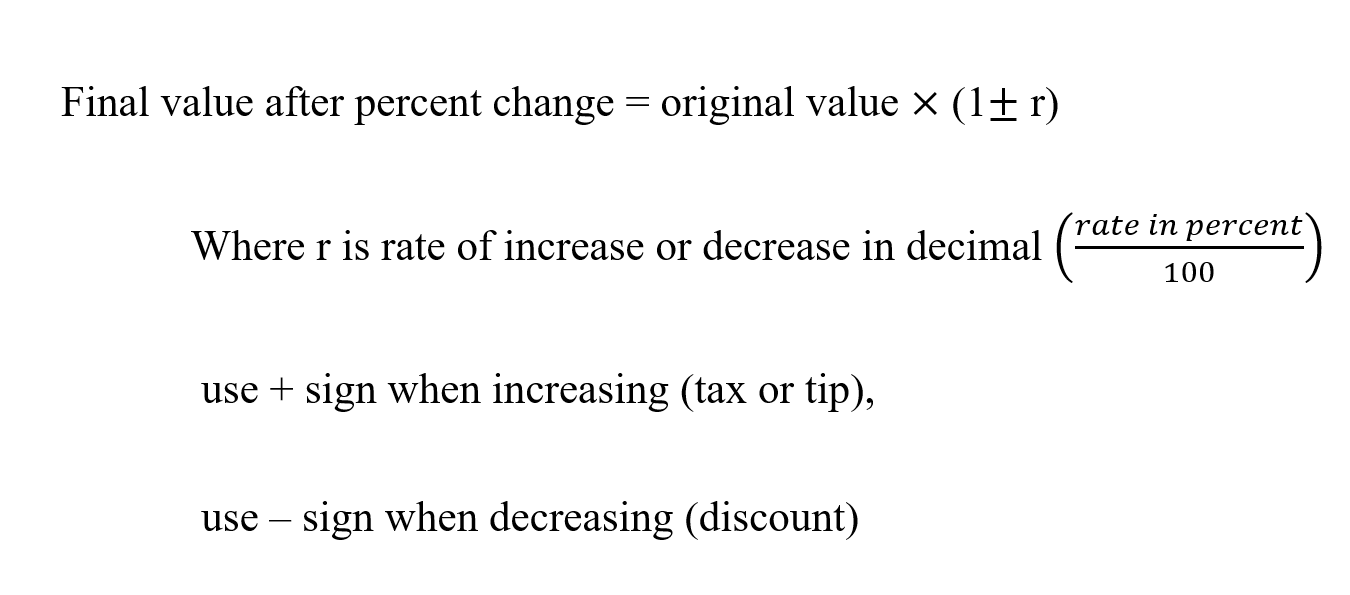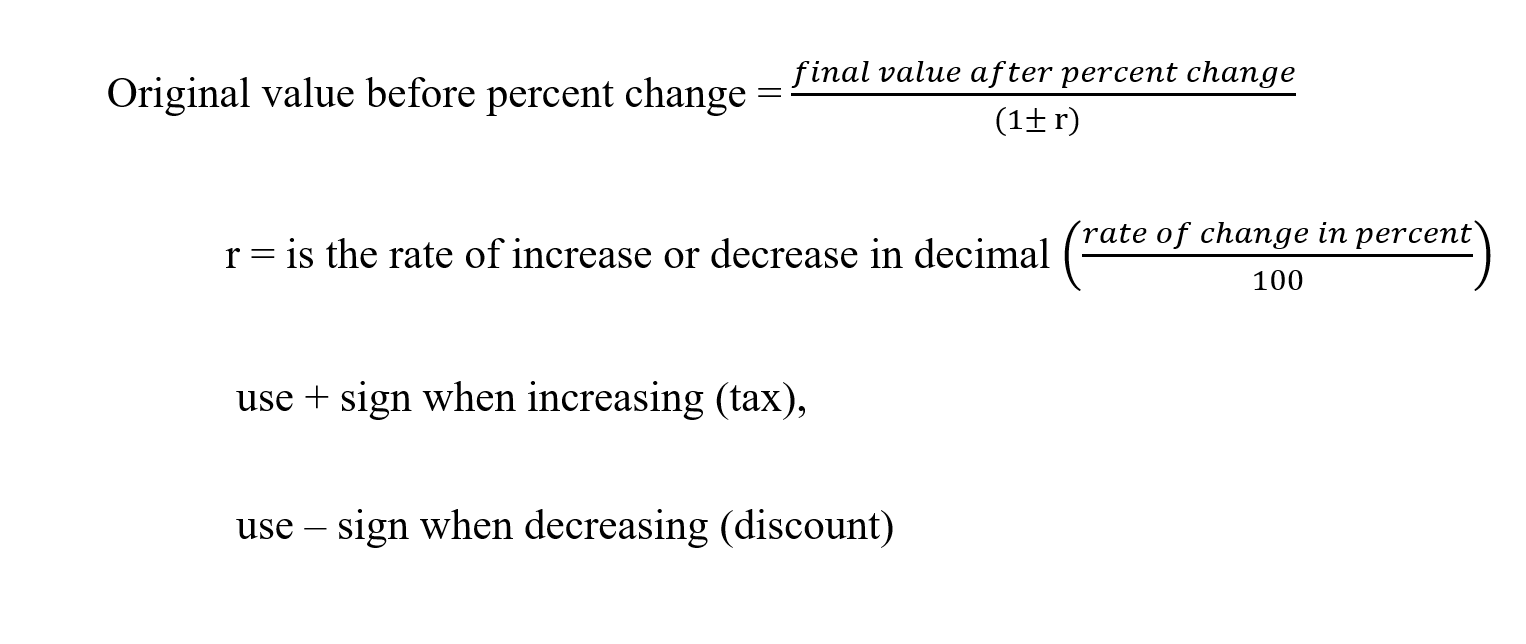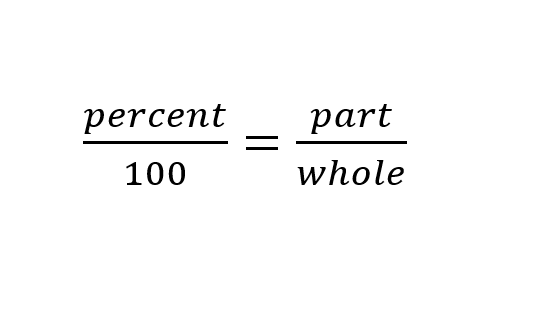Percentage

What is Percentage?

A percentage is a portion of a whole expressed as a number between 0 and 100 rather than as a fraction

How do I find percent change of value?How to find the value after a percent increase of decrease.How to find the original value before a percent increase or decrease?What percent of a number is another number?